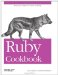# Building a Histogram

Problem

You have an array that contains a lot of references to relatively few objects. You want to create a histogram, or frequency map: something you can use to see how often a given object shows up in the array.

Solution

Build the histogram in a hash, mapping each object found to the number of times it appears.

```	module Enumerable
def to_histogram
inject(Hash.new(0)) { |h, x| h[x] += 1; h}
end
end

[1, 2, 2, 2, 3, 3].to_histogram
# => {1=>1, 2=>3, 3=>2}

["a", "b", nil, "c", "b", nil, "a"].to_histogram
# => {"a"=>2, "b"=>2, "c"=>1, nil=>2}

"Aye
Nay
Nay
Abstaining
Aye
Nay
Not Present
".to_histogram
# => {"Abstaining
"=>1, "Nay
"=>3, "Not Present
"=>1, "Aye
"=>2}

survey_results = { "Alice" => :red, "Bob" => :green, "Carol" => :green,
"Mallory" => :blue }
survey_results.values.to_histogram
# => {:red=>1, :green=>2, :blue=>1}
```

Discussion

Making a histogram is an easy and fast (linear-time) way to summarize a dataset. Histograms expose the relative popularity of the items in a dataset, so they're useful for visualizing optimization problems and dividing the "head" from the "long tail."

Once you have a histogram, you can find the most or least common elements in the list, sort the list by frequency of appearance, or see whether the distribution of items matches your expectations. Many of the other recipes in this book build a histogram as a first step towards a more complex algorithm.

Here's a quick way of visualizing a histogram as an ASCII chart. First, we convert the histogram keys to their string representations so they can be sorted and printed. We also store the histogram value for the key, since we can't do a histogram lookup later based on the string value we'll be using.

```	def draw_graph(histogram, char="#")
pairs = histogram.keys.collect { |x| [x.to_s, histogram[x]] }.sort
```

Then we find the key with the longest string representation. We'll pad the rest of the histogram rows to this length, so that the graph bars will line up correctly.

```	 largest_key_size = pairs.max { |x,y| x.size <=> y.size }.size
```

Then we print each key-value pair, padding with spaces as necessary.

```	 pairs.inject("") do |s,kv|
s << "#{kv.ljust(largest_key_size)} |#{char*kv}
"
end
end
```

Here's a histogram of the color survey results from the Solution:

```	puts draw_graph(survey_results.values.to_histogram)
# blue |#
# green |##
# red |#
```

This code generates a bunch of random numbers, then graphs the random distribution:

```	random = []
100.times { random << rand(10) }
puts draw_graph(random.to_histogram)
# 0 |############
# 1 |########
# 2 |#######
# 3 |#########
# 4 |##########
# 5 |#############
# 6 |###############
# 7 |########
# 8 |#######
# 9 |###########
```

• Recipe 2.8, "Finding Mean, Median, and Mode"
• Recipe 4.9, "Sorting an Array by Frequency of Appearance"Ruby Cookbook (Cookbooks (OReilly))
ISBN: 0596523696
EAN: 2147483647
Year: N/A
Pages: 399